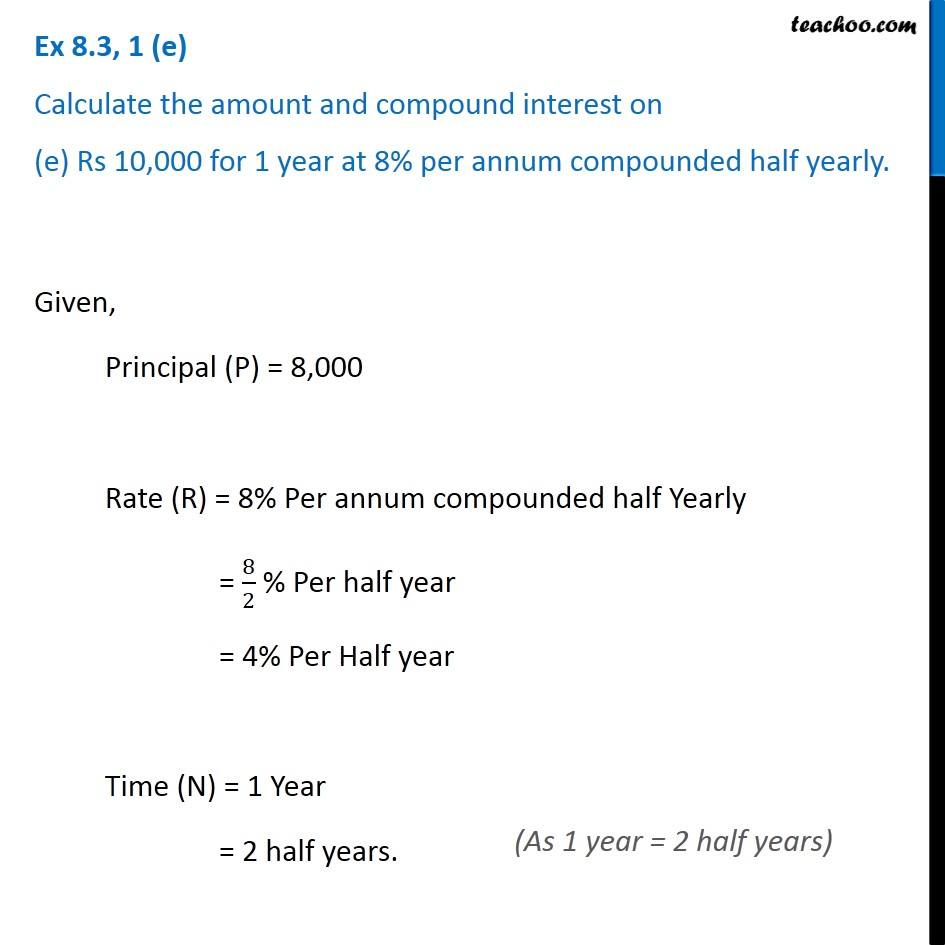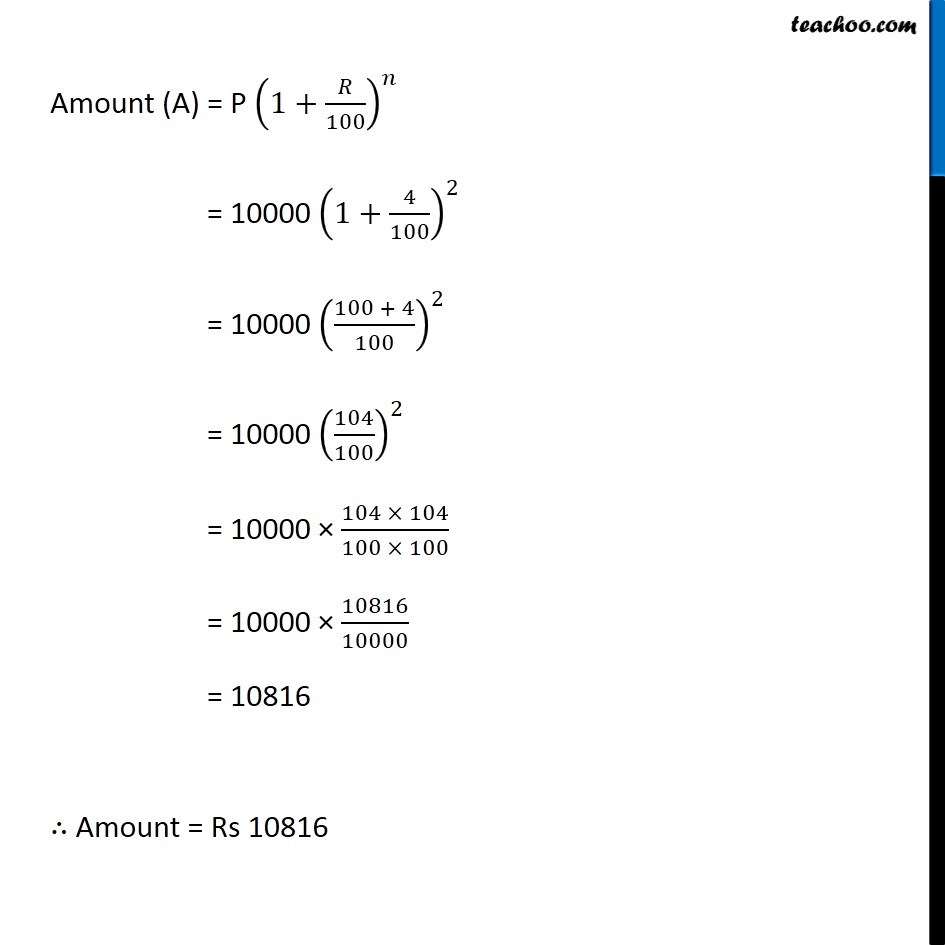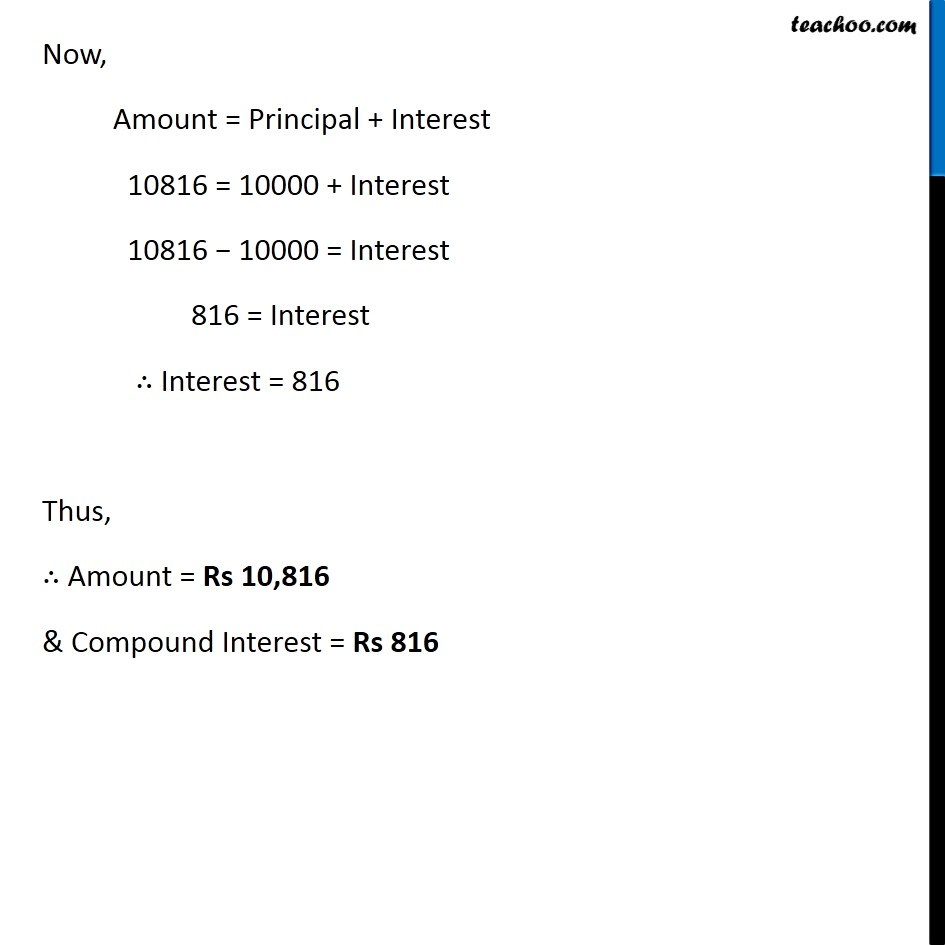Subscribe to our Youtube Channel - https://you.tube/teachoo

1. Chapter 8 Class 8 Comparing Quantities
2. Concept wise
3. Compound Interest compounded half yearly

Transcript

Ex 8.3, 1 (e) Calculate the amount and compound interest on (e) Rs 10,000 for 1 year at 8% per annum compounded half yearly.Given, Principal (P) = 8,000 Rate (R) = 8% Per annum compounded half Yearly = 8/2 % Per half year = 4% Per Half year Time (N) = 1 Year = 2 half years. (As 1 year = 2 half years) Amount (A) = P (1+𝑅/100)^𝑛 = 10000 (1+4/100)^2 = 10000 ((100 + 4)/100)^2 = 10000 (104/100)^2 = 10000 × (104 × 104)/(100 × 100) = 10000 × 10816/10000 = 10816 ∴ Amount = Rs 10816 Now, Amount = Principal + Interest 10816 = 10000 + Interest 10816 − 10000 = Interest 816 = Interest ∴ Interest = 816 Thus, ∴ Amount = Rs 10,816 & Compound Interest = Rs 816

Compound Interest compounded half yearly